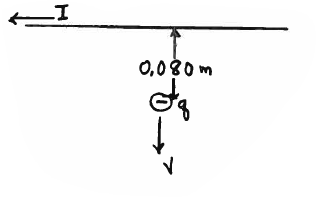# Problem: A long straight wire carries a current of I = 3.00 A directed to the left, as shown in the sketch. A small sphere with negative charge q = -5.00 x 10-4 C is moving from the wire. When the sphere is 0.080 m from the wire, its speed is v = 9.00 m/s. What are the magnitude and direction of the force that the wire exerts on the small sphere when the sphere is at the position shown in the sketch? Write the direction in the blank provided and also show the direction on the sketch. Ans. F = direction =

###### Problem Details

A long straight wire carries a current of I = 3.00 A directed to the left, as shown in the sketch. A small sphere with negative charge q = -5.00 x 10-4 C is moving from the wire. When the sphere is 0.080 m from the wire, its speed is v = 9.00 m/s. What are the magnitude and direction of the force that the wire exerts on the small sphere when the sphere is at the position shown in the sketch? Write the direction in the blank provided and also show the direction on the sketch.

Ans. F =

direction =